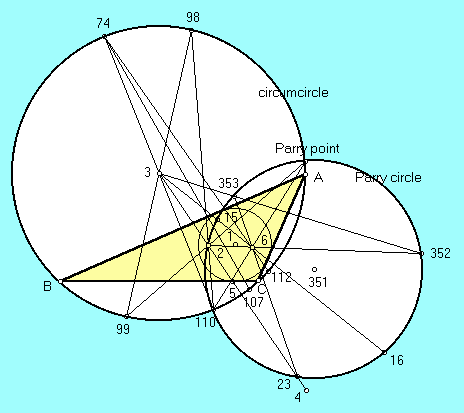# PARRY POINT

 The Parry point is the point of intersection of the circumcircle and the Parry circle. (Defined as the circle passing through the centroid and the two isodynamic points, the Parry circle also passes through several other notable triangle centers.) The Parry circle and Parry point are named in honor of the English geometer Cyril Parry, who studied them in the early 1990s. Trilinears for the Parry point are a/(2 a^2 - b^2 - c^2) : b/(2 b^2 - c^2 - a^2) : c/(2 c^2 - a^2 - b^2).(For a quick lesson on trilinears, click on TRILINEAR COORDINATES.)

Triangle Centers